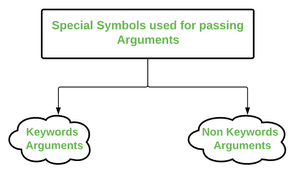# *args and **kwargs in Python

In Python, we can pass a variable number of arguments to a function using special symbols. There are two special symbols:Special Symbols Used for passing arguments:-

1.)*args (Non-Keyword Arguments)

2.)**kwargs (Keyword Arguments)

“We use *args and **kwargs as an argument when we have no doubt about the number of  arguments we should pass in a function.”

1.) *args

The special syntax *args in function definitions in python is used to pass a variable number of arguments to a function. It is used to pass a non-key worded, variable-length argument list.

• The syntax is to use the symbol * to take in a variable number of arguments; by convention, it is often used with the word args.
• What *args allows you to do is take in more arguments than the number of formal arguments that you previously defined. With *args, any number of extra arguments can be tacked on to your current formal parameters (including zero extra arguments).
• For example : we want to make a multiply function that takes any number of arguments and able to multiply them all together. It can be done using *args.
• Using the *, the variable that we associate with the * becomes an iterable meaning you can do things like iterate over it, run some higher-order functions such as map and filter, etc.

## python3

 `# Python program to illustrate  ` `# *args for variable number of arguments` `def` `myFun(``*``argv): ` `    ``for` `arg ``in` `argv: ` `        ``print` `(arg)` `  `  `myFun(``'Hello'``, ``'Welcome'``, ``'to'``, ``'GeeksforGeeks'``) `

Output:

```Hello
Welcome
to
GeeksforGeeks

```

## Python3

 `# Python program to illustrate ` `# *args with first extra argument` `def` `myFun(arg1, ``*``argv):` `    ``print` `(``"First argument :"``, arg1)` `    ``for` `arg ``in` `argv:` `        ``print``(``"Next argument through *argv :"``, arg)`   `myFun(``'Hello'``, ``'Welcome'``, ``'to'``, ``'GeeksforGeeks'``)`

Output:

```First argument : Hello
Next argument through *argv : Welcome
Next argument through *argv : to
Next argument through *argv : GeeksforGeeks

```

2.)**kwargs

The special syntax **kwargs in function definitions in python is used to pass a keyworded, variable-length argument list. We use the name kwargs with the double star. The reason is because the double star allows us to pass through keyword arguments (and any number of them).

•  A keyword argument is where you provide a name to the variable as you pass it into the function.
• One can think of the kwargs as being a dictionary that maps each keyword to the value that we pass alongside it. That is why when we iterate over the kwargs there doesn’t seem to be any order in which they were printed out.

Example for usage of **kwargs:

## python

 `# Python program to illustrate  ` `# *kargs for variable number of keyword arguments`   `def` `myFun(``*``*``kwargs): ` `    ``for` `key, value ``in` `kwargs.items():` `        ``print` `(``"%s == %s"` `%``(key, value))`   `# Driver code` `myFun(first ``=``'Geeks'``, mid ``=``'for'``, last``=``'Geeks'``)    `

Output:

```last == Geeks
mid == for
first == Geeks

```

## python

 `# Python program to illustrate  **kargs for ` `# variable number of keyword arguments with` `# one extra argument.`   `def` `myFun(arg1, ``*``*``kwargs): ` `    ``for` `key, value ``in` `kwargs.items():` `        ``print` `(``"%s == %s"` `%``(key, value))`   `# Driver code` `myFun(``"Hi"``, first ``=``'Geeks'``, mid ``=``'for'``, last``=``'Geeks'``)    `

Output:

```last == Geeks
mid == for
first == Geeks

```

Example:

## python3

 `def` `myFun(arg1, arg2, arg3):` `    ``print``(``"arg1:"``, arg1)` `    ``print``(``"arg2:"``, arg2)` `    ``print``(``"arg3:"``, arg3)` `    `  `# Now we can use *args or **kwargs to` `# pass arguments to this function : ` `args ``=` `(``"Geeks"``, ``"for"``, ``"Geeks"``)` `myFun(``*``args)`   `kwargs ``=` `{``"arg1"` `: ``"Geeks"``, ``"arg2"` `: ``"for"``, ``"arg3"` `: ``"Geeks"``}` `myFun(``*``*``kwargs)`

Output:

```arg1: Geeks
arg2: for
arg3: Geeks
arg1: Geeks
arg2: for
arg3: Geeks

```

Example:

## python3

 `def` `myFun(``*``args,``*``*``kwargs):` `    ``print``(``"args: "``, args)` `    ``print``(``"kwargs: "``, kwargs)`     `# Now we can use both *args ,**kwargs to pass arguments to this function :` `myFun(``'geeks'``,``'for'``,``'geeks'``,first``=``"Geeks"``,mid``=``"for"``,last``=``"Geeks"``)`

Output:

```args: ('geeks', 'for', 'geeks')
kwargs {'first': 'Geeks', 'mid': 'for', 'last': 'Geeks'}

```

This article is contributed by Kishlay Verma. If you like GeeksforGeeks and would like to contribute, you can also write an article using contribute.geeksforgeeks.org or mail your article to contribute@geeksforgeeks.org. See your article appearing on the GeeksforGeeks main page and help other Geeks.